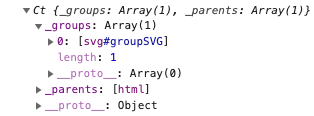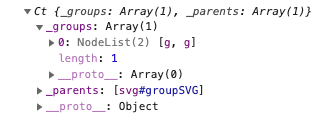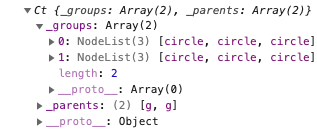# Chapter 01Creating Selection

When creating data visualizations we often need to apply a set of visual changes to a set of elements in the DOM. Sometimes we can retrieve the set we need by calling `getElementsByClassName`, `getElementsByTagName`, and `getElementById`. But often times, these are insufficient. D3.js has an incredibly useful and versatile module named Selections that allow us to select sets of elements from the DOM using CSS selectors and user-defined functions.

The D3 API has 3 initial methods that can be used to select sets of elements from the DOM. These are:

Each one of the methods above return a d3.selection object containing zero or more element nodes from the DOM. Once we have a selection object we can call the various selection methods to manipulate the DOM as we’ll see in the next section.

d3.select(selector) returns a selection containing zero or one element or node. The `selector` argument can be either an string that holds any valid CSS selector or a reference to a node. If the selector is a string, it returns a selection containing the first element found in the DOM that meets the CSS selector criteria.  If no element is found then it returns an empty selection.  If the selector is a reference to a node (e.g. this) then it returns a section object containing that node.

d3.selectAll(selector) returns a selection of zero or more elements or nodes. The method takes as an argument a string that holds a CSS selector, an array of nodes, or a pseudo-array like a `NodeList`.  If the argument is a string, the method returns a selection containing all of the elements that match the CSS selector defined by the string.  If no elements match, the selection object that is returned is empty.  If the selector contains references to nodes then the method returns a selection that contains the nodes.

d3.selection() is used to retrieve a selection object that only contains the root document element (i.e. document.documentElement) of the web page. This method can can also be used to test for the `selection` type using `instanceof d3.selection`.

To illustrate various ways that we can use these methods, let’s suppose we have 5 circles rendered in an SVG as shown below.

```<svg width="425" height="100" >
<circle class="lightblue" cx="50" cy="50" r="25" />
<circle class="lightblue" cx="125" cy="50" r="25" />
<circle class="pink" cx="200" cy="50" r="25" />
<circle id="secondPink" class="pink" cx="275" cy="50" r="25"" />
<circle cx="350" cy="50" r="25" fill="aquamarine" />
</svg>```

In the script below we select different subsets of the 5 circles using the selection methods. Note that we don’t do anything with the selections just yet; we’re just demonstrating how to select sets of elements.

```let firstBlueCircle = d3.select(".lightblue");
let allBlueCircles = d3.selectAll(".lightblue");
let secondPinkCircle = d3.select("#secondPink");
let allCircles = d3.selectAll("circle");
```

In the first statement we set `firstBlueCircle` equal to the selection object that contains a single element, the first element having the class name `lightblue`. The second statement uses the same CSS selector, but calls `selectAll` to return a selection containing all of the elements having a class name `lightblue`. The third statement sets the variable `secondPinkCircle` to the selection object containing a single element, namely the element whose id attribute is set to `secondPink`. The fourth statement retrieves a selection containing all of the `circle` elements in the web page’s DOM.

Remember that each of the select methods discussed above return a selection object, not a Node object or a set of Node objects. Also, any valid CSS selector can be passed to `select` and `selectAll`.

## Chaining Method Calls

The `d3.selection` type has many methods that return a new selection object. As such, we can chain multiple `selection` method calls together in a single statement.

In the example below, `selectAll` and `attr` return selection objects, so rather than writing code to set the `r` and `fill` properties of a set of circle elements like this:

```let sel = d3.selectAll("circle");
sel.attr("r", "30");
sel.attr("fill", "pink");
```

We can, instead, chain the method calls together and write an equivalent statement like the one below.

```  d3.selectAll("circle")
.attr("r", "30")
.attr("fill", "pink");
```

## Selecting Descendant Elements

The `d3.selection` type has two methods that allow you to select zero or more descendant elements based on the elements in the current selection.  Per the API, these methods are:

Both `selection.select` and `selection.selectAll` have one argument, a selector. The value passed in can be either a string or a selector function. If the argument is a string, it is interpreted as a CSS selector. If the argument is a function then the function is called for each element of the selection. When the selector function is called for an element of the selection, the function is passed 3 values: (`d`,`i`,`nodes`) where `d` holds data that might have been joined to the element, `i` holds the index of the element, and `node`s is a reference to a NodeList holding the element. With this data we can retrieve the element for which the function is called using `nodes[i]`.

If a selector function is passed to `selection.select`, the selector function must return a single element of null. If a selector function is passed to `selection.selectAll`, the selector function must return an array or pseudo-array, like a NodeList.

Consider the SVG in the example below. The SVG element uses `&lt;g&gt;` tags to group circle elements into rows. In order to get all of the circles that are descendants of the first `g` element we call `selection.select` to get the first `g` element and then chain a call to `selection.selectAll` to get all of the `circle` elements that are children of the selected `g` element. Once we have a selection containing the elements we want to modify, we can modify the attributes of the elements in the selection using the `attr` method. Note: we discuss the `attr` method in the next section.

```d3.select("g")
.selectAll("circle")
.attr("stroke", "gray")
.attr("stroke-width", "3");
```
```<script>
function nestedSelect() {
d3.select("g")
.selectAll("circle")
.attr("stroke", "gray")
.attr("stroke-width", "3");
}
</script>

<svg width="160" height="110">
<g>
<circle r="20" cx="30" cy="30" fill="lightblue" />
<circle r="20" cx="80" cy="30" fill="lightblue" />
<circle r="20" cx="130" cy="30" fill="lightblue" />
</g>
<g>
<circle r="20" cx="30" cy="80" fill="lightblue" />
<circle r="20" cx="80" cy="80" fill="lightblue" />
<circle r="20" cx="130" cy="80" fill="lightblue" />
</g>
</svg>

## Groups

In this section we discuss how D3.js stores elements in a selection object. When doing so, we’ll refer to the following example which calls `d3.select` and `d3.selectAll` in order to select all of the circle elements in the SVG.

```<script>
var a = d3.select("#groupSVG")
var b = a.selectAll("g");
var c = b.selectAll("circle")
.attr("stroke", "gray")
.attr("stroke-width", "3");
</script>

<svg id="groupSVG" width="160" height="110">
<g>
<circle r="20" cx="30" cy="30" fill="pink" />
<circle r="20" cx="80" cy="30" fill="pink" />
<circle r="20" cx="130" cy="30" fill="pink" />
</g>
<g>
<circle r="20" cx="30" cy="80" fill="pink" />
<circle r="20" cx="80" cy="80" fill="pink" />
<circle r="20" cx="130" cy="80" fill="pink" />
</g>
</svg>```

If you inspect a selection object in the browser’s console you’ll notice that a selection object contains a `_parents` property and a `_groups` property. The `_parents` property contains an array of Nodes, which coorespond to the elements from which the search for selected elements takes place. When we use `d3.select` or `d3.selectAll` we are searching from the document’s `html` element, thus we see that the `_parents` property holds an array containing a single element, the `html` node.

In our example, the variable `a` holds a selection that was returned by `d3.select("#groupSVG")`. Since we use `d3.select` we search the entire document starting at the `html` element. Thus, as stated above, the selection’s `_parents` property contains an array holding the document’s `html` node.The `_groups` property contains an array of `NodeList` objects, each holding a group of element nodes that are descendants of a parent node. The number of NodeLists will always be equal to the number of parents and `_groups[i]` will be the NodeList holding the descendants of `_parents[i]`.

When we call `d3.select` or `d3.selectAll`, since there is only one parent node, the document’s `html` node, we’ll find there is ever only one NodeList element in the `_groups` array.

In our example, selection `a` has one parent (the document’s `html` element), thus it also has only one NodeList in `_groups` and that NodeList contains the SVG element that was selected.

Now, when we call `select` or `selectAll` on a selection, all of the elements in the selection’s NodeLists become parents in the new selection and as before, there will be one NodeList in the selection’s `_groups` array for each parent.

So, for example, when we select the g elements that are descendants of the SVG by calling `b = a.selectAll("g")` the elements in `a's` NodeLists become the parents in `b`. Since the total number of elements the `a's` NodeLists is 1, then there is only one parent in `b's` `_parents` array (the SVG element) and one NodeList containing the two g elements that are descendants of the SVG element.The second call to `selectAll` selects all of the circle elements that are descendants of the g elements in `b`. Since there are two g elements in `b` there are two parents in `c` with each parent being assigned a `NodeList` containing the `circle` elements that are descendants of their parent `g` elements.For more information on groups and how selections are created we recommend you read Bostock’s article titled How Selections Work.

## Filtering Selections

The selection.filter(filter) method takes a filter as an argument and returns a selection containing a subset of the objects in the selection on which it is called. The `filter` argument can be either a selection string as discussed above or a function. If the filter is a selection string, the method returns the elements in the selection that match the selection string.

If the filter is a function, the function is called for each element in the selection, in order. The elements for which the function returns true are retained in the selection and the others are removed. When the function is called for an element, the function is passed three arguments (d, i, nodes): the datum joined to the element (discussed in the next chapter), an integer specifying the group index of the element, and the group itself. As you can see in the example code, the filter method uses `nodes[i]` to access the element in the selection that is being processed.

In the example below, the 5 circle elements have radii between 5 and 25.  We select all of the circle elements, filter the selection down to only those with radii greater than or equal to 20, then remove the elements left in the selection.

```<script>
.filter((d,i,nodes) => nodes[i].getAttribute("r") >= 20)
.remove();
}
</script>

<circle cx="50" cy="50" r="5" fill="pink" />
<circle cx="125" cy="50" r="10" fill="pink" />
<circle cx="200" cy="50" r="15" fill="pink" />
<circle cx="275" cy="50" r="20" fill="pink" />
<circle cx="350" cy="50" r="25" fill="pink" />
</svg>```

Note that `selection.filter` preserves the parents of the `selection` on which it is called, but does not preserve the indexes of the remaining elements in groups `NodeLists`.

## Auxiliary Functions

D3.js contains three generator functions that are used by `selection.filter`, `selection.select`, and `selection.selectAll`.

• d3.matcher(selector) - returns a function that when run returns true if this matches the selector passed in as an argument.

• d3.selector(selector) - returns a function that when run returns the first descendant of this that matches the selector passed in as an argument.

• d3.selectorAll(selector) - returns a function that when run returns a `NodeList` containing the descendant of this that matches the selector passed in as an argument.

Below we show examples of how `d3.matcher` and `d3.selector` can be used. By calling `call` on each of the functions that are generated (`matcher` and `selector`) we change the execution context of the functions thus changing the value of `this` in each.

```<script>
var div = document.createElement('div');
div.id = "auxDiv";
document.body.appendChild(div);

var matcher = d3.matcher('div');
var selector = d3.selector('#auxDiv');

console.log(matcher.call(div));
console.log(selector.call(document.body).id);
</script>```